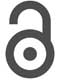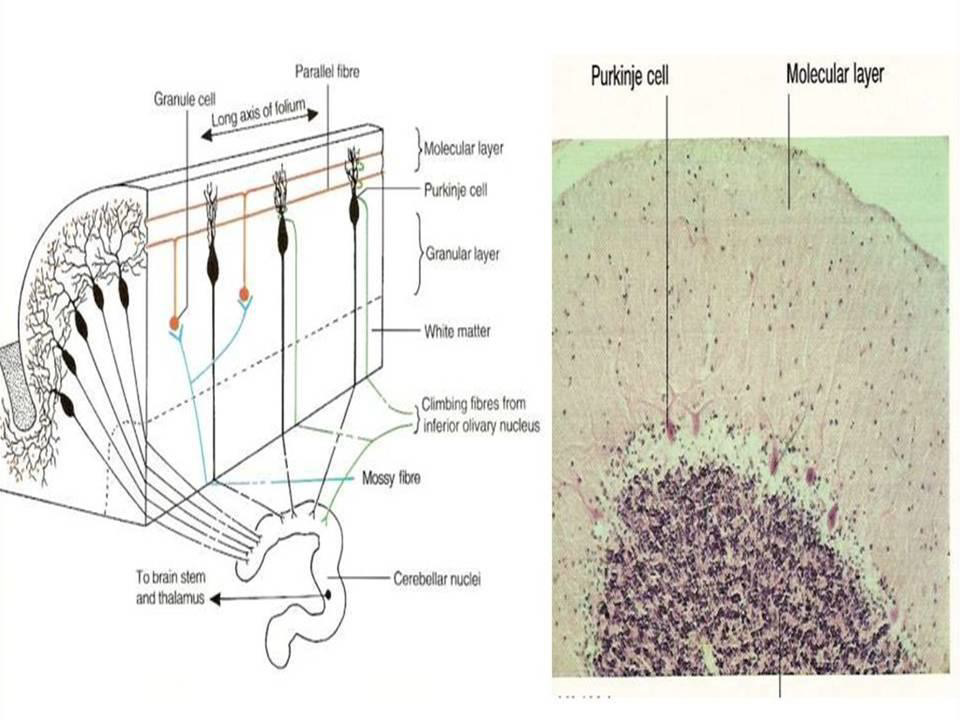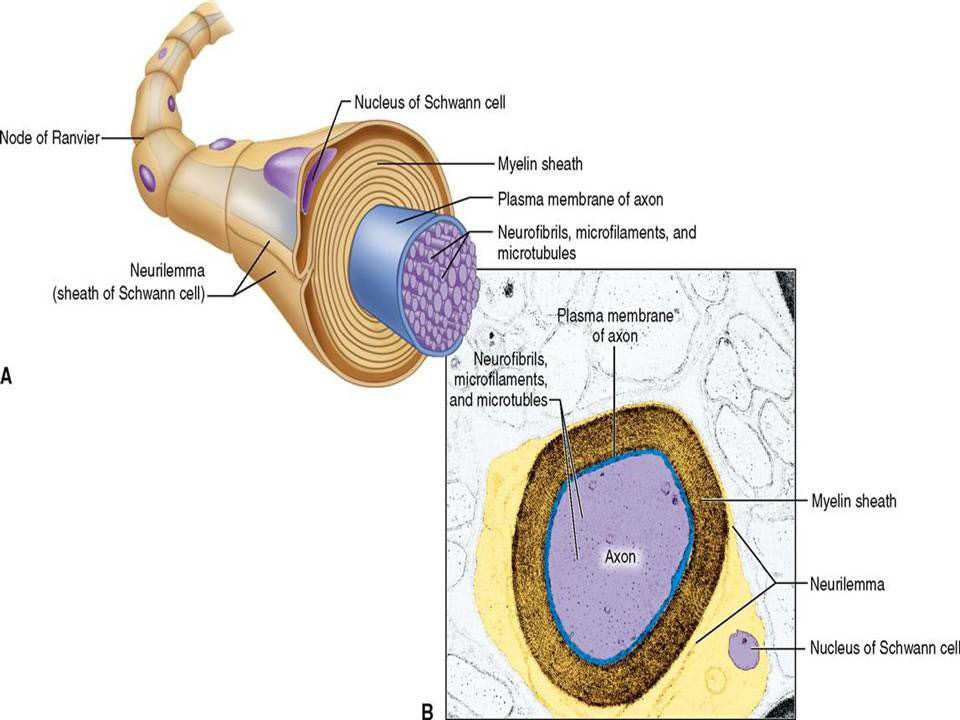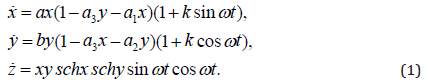## Volume 14 - Issue 3

Review ArticleCreative Commons, CC-BY

# The Use of Multilayer Mesh Electrically Conductive Nanotubes Taking into Account Chirality in Damaged Active and Inactive Neural Networks

*Corresponding author: Sergey Belyakin, Department of General Physics, Physics Faculty, Lomonosov Moscow State University, Moscow, Russia.

Received: September 08, 2021; Published: September 28, 2021

#### Abstract

In this article, we will consider mesh multilayer nanotubes for axon modeling. For modeling, we use a dynamic soliton model that takes into account the chirality conditions in active and passive neural networks. Based on this model, it is supposed to study the state of the network. The term neural networks refers to the networks of neurons in the mammalian brain. Neurons are its main units of computation. In the brain, they are connected together in a network to process data. This can be a very complex task, and so the dynamics of neural networks in the mammalian brain in response to external stimuli can be quite complex. The inputs and outputs of each neuron change as a function of time, in the form of so-called spike chains, but the network itself also changes. We learn and improve our data processing capabilities by establishing reconnections between neurons [1-3]. The training set contains a list of input data sets along with a list of corresponding target values that encode the properties of the input data that the network needs to learn. To solve such associative problems, artificial neural networks can work well, when new data sets are governed by the same principles that gave rise to the training data .

Keywords: Nonlinear Dynamic System; Soliton; Chirality; Neural Networks; Neuron; Axon; Nanotubes

#### Neural Networks

The mammalian brain is made up of different areas that perform different tasks. The cortex is the outer layer of the mammalian brain. We can think of it as a thin sheet (2 to 5 mm thick) that folds on its own to increase its surface area. The cerebral cortex is the largest and most developed part of the human brain. It contains a large number of nerve cells, neurons. The human cortex contains about 1010 neurons. They are connected by nerve threads (axons) that branch out and end in synapses. These synapses are connections to other neurons. Synapses connect to dendrites, branched extensions of the body of a nerve cell designed to receive input signals from other neurons in the form of electrical signals. A neuron in the human brain can have thousands of synaptic connections to other neurons. The resulting network of connected neurons in the cortex is responsible for processing visual, audio, and sensory data. (Figure 1) Neurons in the cerebral cortex (the outer layer of the brain, the largest and most developed part of the human and mammalian brain). (Figure 2) shows a more schematic view of the neuron. The information is processed from left to right. On the left are the dendrites that receive the signals and connect to the cell body of the neuron, where the signal is processed. The right part of the picture shows the axon through which the output is directed to the dendrites of other neurons. The information is transmitted as an electrical signal. The information is transmitted as an electrical signal (Figure 3) shows a Schwann cell, which can be in a neutral state and create a left positive or right negative chirality on the axon. (Figure 4) shows an example of the time series of the electric potential of a pyramidal neuron . The time series consists of an intermittent series of electric potential jumps. Periods of rest without spikes occur when the neuron is inactive, and during periods rich in spikes, the neuron is active. (Figure 4D) Temporary portraits of the system (1) (Figure 5).Figure 1: Neurons in the cerebral cortex (the outer layer of the brain, the largest and most developed part of the human and mammalian brain).Figure 2: Schematic representation of a neuron. Dendrites receive input signals in the form of electrical signals through synapses. The signals are processed in the cell body of the neuron. The output signal is transmitted from the body of the nerve cell to other neurons via the axon.Figure 3: Shows a Schwann cell, which can be in a neutral state and create a left positive or right negative chirality on the axon.Figure 4: Shows an example of the time series of the electric potential of a pyramidal neuron. The time series consists of an intermittent series of electric potential jumps.

#### Mesh Nanotubes

##### The mathematical dynamic model of the soliton

The mathematical dynamic model of the soliton is represented by the equation (1) [6,7].Time portraits of the system (1) are shown in (Figure6a) active with positive

a =1.0, a1 = 0.2, a2 = 0.1, a3 = 1.0, b = –2.0, k = 0.045, ω = 64π and negative a =–1.0, a1 = 0.2, a2 = –0.1, a3 = 1.0, b = 2.0, k = 0.045, ω = 64π chirality. (Figure 6b) passive positive a =0.09, a1 = 0.2, a2 =0.1, a3 = 1.0, b = –0.09, k = 0.045, ω = 64π and negative a =–0.09, a1 = 0.2, a2 = –0.1, a3 = 1.0, b = 0.09, k = 0.045, ω = 64π chirality: Time portraits of the system (1) are shown in (Figure 7a) active with positive a =1.0, a1 = 0.2, a2 = 0.1, a3 = 1.0, b = 2.0, k = 0.045, ω = 64π and negative a =–1.0, a1 = 0.2, a2 = –0.1, a3 = 1.0, b = –2.0, k = 0.045, ω = 64π lack of chirality. (Figure 7b) passive positive a =0.09, a1 = 0.2, a2 =0.1, a3 = 1.0, b = 0.09, k = 0.045, ω = 64π and negative a =–0.09, a1 = 0.2, a2 = –0.1, a3 = 1.0, b =– 0.09, k = 0.045, ω = 64π lack of chirality:Figure 6: Temporary portraits of the system with chirality. (1) at : a) x0 = 0.4, y0 = 0.4, z0 = 2.5, b) x0 = 0.4, y0 = 0.4, z0 = 0.09.Figure 7: Temporary portraits of the system with no chirality. (1) at : a) x0 = 0.4, y0 = 0.4, z0 = 2.5, b) x0 = 0.4, y0 = 0.4, z0 = 0.09.

#### Summary

Artificial neural networks use a highly simplified model for the fundamental computing unit-the neuron. In its simplest form, the model is simply a binary threshold unit. The network performs these calculations sequentially. Usually, discrete sequences of calculation time steps are considered, t = 0,1,2,3.... Either all neurons are updated simultaneously at one time step (synchronous update), or only one selected neuron is updated (asynchronous update) [8-19]. We proposed a different approach to use the wave-soliton approach, taking into account chirality. In the presence of different chirality, three states are possible, with positive chirality, the signal is transmitted without loss, with negative chirality, the signal creates pulsations in certain parts of the axon. In the absence of a signal, a chaotic self-excitation is observed in the axon. A run a way with no chirality exists for a short time and represents a stop signal. The conclusion is that the soliton model can be used to study the behavior of an individual axon , and the damaged sections of axons can be replaced by multilayer mesh electro-conducting nanotubes.